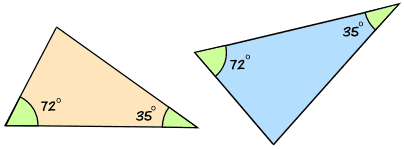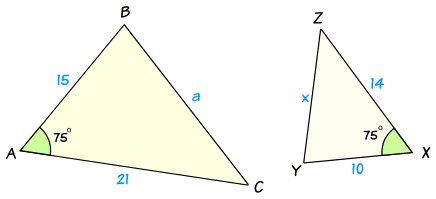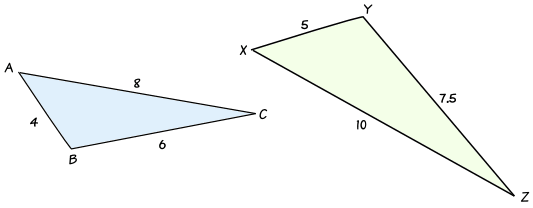# 相似三角形的判定

• 对应角（同位角）相等，
• 对应边成比例比

## AA

AA 代表 "角、角"，英语 "angle, angle"，意思是两个三角形有两个角是相同的。

### 例子：这两个三角形是相似的：## SAS

SAS 代表 "边、角、边"，英语 "side, angle, side"，意思是在两个三角形里：

• 两组对应边成比例
• 对应的夹角相等。

### 例子：• 一对边的比是 21 : 14 = 3 : 2
• 另一对边的比是 15 : 10 = 3 : 2
• 两条边之间的角在两个三角形里都是 75°

### 例子（续）

• a2 = b2 + c2 - 2bc cos A
• a2 = 212 + 152 - 2 × 21 × 15 × Cos75°
• a2 = 441 + 225 - 630 × 0.2588……
• a2 = 666 - 163.055……
• a2 = 502.944……
• 所以 a = √502.94 = 22.426……

• x2 = y2 + z2 - 2yz cos X
• x2 = 142 + 102 - 2 × 14 × 10 × Cos75°
• x2 = 196 + 100 - 280 × 0.2588……
• x2 = 296 - 72.469……
• x2 = 223.530……
• 所以 x = √223.530…… = 14.950……

a : x = 22.426^ : 14.950^ = 3 : 2

## SSS

SSS 代表 "边、边、边"，英语 "side, side, side"，意思是两个三角形的三组对应边成比例。

### 例子：• a : x = 6 : 7.5 = 12 : 15 = 4 : 5
• b : y = 8 : 10 = 4 : 5
• c : z = 4 : 5

### 用三角学

• cos A = (b2 + c2 - a2)/2bc
• cos A = (82 + 42 - 62)/(2× 8 × 4)
• cos A = (64 + 16 - 36)/64
• cos A = 44/64
• cos A = 0.6875
• 所以角A = 46.6°

• cos X = (y2 + z2 - x2)/2yz
• cos X = (102 + 52 - 7.52)/(2× 10 × 5)
• cos X = (100 + 25 - 56.25)/100
• cos X = 68.75/100
• cos X = 0.6875
• 所以角X = 46.6°

A 和 X 是相等的！